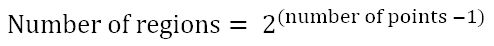Search

# Assumptions can be helpful - and dangerous

Updated: Nov 22, 2020People, and machines, are great at generalising. We look at a number of specific instances and use reasoning to transfer what we have learned to a new example. This is a valuable skill - as long as we take care. Things are not always as they appear! Let's look at a particularly interesting case.

In the diagram below there are five circles. In circle A, we draw 2 non-coincident points on it's circumference and connect them with a line. This creates 2 regions.In circle B we draw 3 points and connect them to create 4 regions. Connecting 4 points in circle C gives us 8 regions. And finally, for circle D we connect 5 points which creates 16 regions.

There is a pattern developing here. For each circle, the following formula seems to provide a way to calculate the number of regions from the number of points.Circle E is our last part of the experiment and here we connect 6 points together. Can we assume that the number of regions is given by our formula above? In reality, there are 31 regions in circle E, not 32 as our formula predicts. The actual equation to calculate the number of regions given the number of points is:Where p is the number of points on the circumference of the circle.

The extent to which this may get you into trouble will depend on what you are doing. For example, Newtonian mechanics is often a valid approximation for Einstein's relativistic mechanics. Newton's equations have been sufficient to design space rockets even though we know there are limits to these equations as speed and mass increase. Treating Pi as 22/7 may be valid in some cases and completely invalid in others. The example here has very limited cases where our initial assumption is true.

In mathematical terms, a conjecture is a conclusion or proposition which is suspected to be true due to preliminary supporting evidence, but for which no general proof or disproof has yet been found. If a conjecture is proven, it then becomes a theorem. It's interesting because using these definitions Fermat's last theorem should actually have been called Fermat's last conjecture. At least until Andrew Wiles provided a proof in 1993.

Do you need to inject a little creative thinking into your business? Please click below to find out more.

21 views